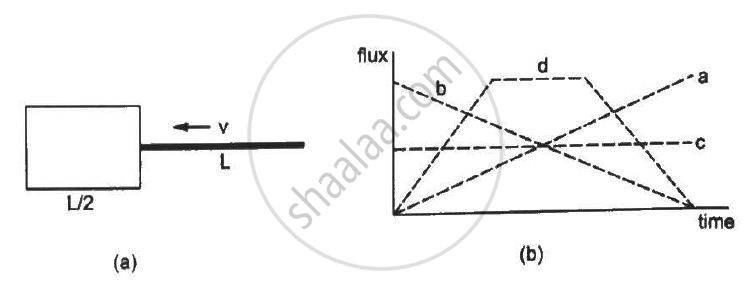Department of Pre-University Education, Karnataka course PUC Karnataka Science Class 12
Share

# Following Figure (A) Shows an Imaginary Cube of Edge L/2. a Uniformly Charged Rod of Length (L) Moves Towards the Left at a Small but Constant Speed - Physics

ConceptElectric Flux

#### Question

Following Figure (a) shows an imaginary cube of edge L/2. A uniformly charged rod of length (L) moves towards the left at a small but constant speed nu. At t = 0, the left end just touches the centre of the face of the cube opposite it. Which of the graphs shown in the figure (b) represents the flux of the electric field through the cube as the rod goes through it?• a

• b

• c

• d

#### Solution

(d)

At first, when the rod is inserted into the cube, the flux start increasing. When the rod is fully inserted, the flux becomes constant and remains constant for the remaining L/2 length of the rod. After that, as the rod moves out of the cube, the flux starts decreasing. These processes are depicted only by curve (d).

Is there an error in this question or solution?

#### APPEARS IN

HC Verma Solution for Concepts of Physics - Vol. 2 (2018 to Current)
Chapter 8: Gauss’s Law
MCQ | Q: 6 | Page no. 140

#### Video TutorialsVIEW ALL 

Solution Following Figure (A) Shows an Imaginary Cube of Edge L/2. a Uniformly Charged Rod of Length (L) Moves Towards the Left at a Small but Constant Speed Concept: Electric Flux.
S